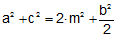Median length, Apollonius' Theorem The following theorem has been attributed to Apollonius of Perga: In any triangle, the sum of the squares on any two sides is equal to twice the square on half the third side together with twice the square on the median which bisects the third side. In other words, if BM is a median of a triangle ABC and if AB = c, BC = a, AC = b, and BM = m, prove that.Home | Geometry | Search | Problems | Apollonius of Perga | Euclid's Elements | Classical Theorems | Email | By Antonio Gutierrez Aug 23, 2014